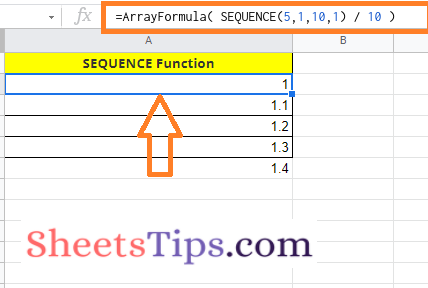# How To Use Sequence Function To Build Numbered List In Google Sheets?

There are multiple methods with the help of which we can easily build numbered lists in Google Sheets. But when we are using the Sequence function to build numbered lists, our work gets easier. Just by entering the Sequence function formula in Google Sheets, we generate various types of number lists such as ascending numbers, 2 dimensional numbers, descending numbers, and so on. However, to generate all these numbers, we need to tweak the Sequence function formula which is discussed in detail on this page. Read further to know how to generate the sequence numbers using the Google Sheets Tips. Read further to find more.

## Sequence Function Syntax in Google Sheets

The syntax or formula to use the sequence function syntax are explained below:

=SEQUENCE(rows, columns, start, step)

• Rows: The number of rows that should be returned.
• Columns: The number of columns that should be returned. If this parameter is missing, the returned array will only have one column.
• Start: The number at which the series should begin. If this parameter is left blank, the series will begin at 1.
• Step:  The amount by which each number in the sequence should be increased or decreased. If this parameter is left out, the sequence will be increased by one.

### How To Use Sequence Function in Google Sheets?

We can generate various types of number lists using the Sequence function in Google Sheets. Let us see some of the examples with the help of which we can generate the numbered lists in Google Sheets.

### Generating Numbered List Using Sequence Function in Google Sheets

The steps to generate the numbered list in Google Sheets using the Sequence function are as follows:

• 1st Step: Open the Google Spreadsheet where you want to generate the Sequence numbers.
• 2nd Step: Now move to the cell where you want to generate the numbered list.
• 3rd Step: Enter the formula “=sequence(4)“.
• 4th Step: Press the “Enter” button and you will see the results as shown below.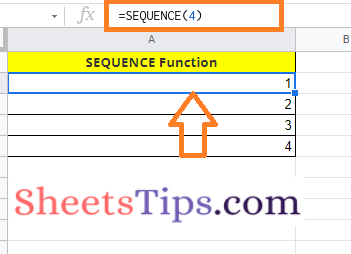Here we have used the number 4. If we have used the number sequence as 5, then 5 sequences of numbers will be generated.

### Generating Horizontal Number List Using Sequence Function in Google Sheets

The steps to generate the horizontal number list in Google Sheets using the sequence function are as follows:

• 1st Step: Open the Google Sheets where you want to generate the horizontal numbered list.
• 2nd Step: Now on the homepage, move to the cell where you want to generate the numbered list.
• 3rd Step: Here enter the formula =Sequence(1,5).
• 4th Step: Press the “Enter” button and you will see the results as shown below.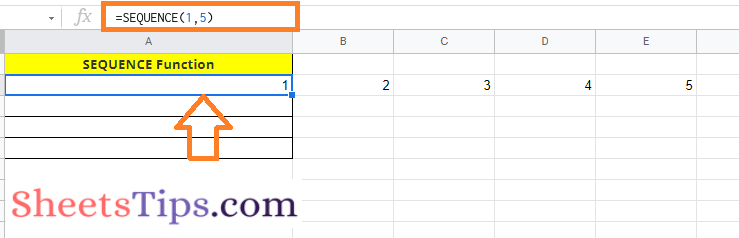### Generating Two-dimensional Array Numbers Using Sequence Function in Google Sheets

We can also easily generate the 2 dimensional array numbers in Google Sheets using the Sequence functions. The steps to get this done are as follows:

• 1st Step: Open the Google Sheets where you want to generate the Two Dimensional Array.
• 2nd Step: Now on the homepage, move to the cell where you want to generate the 2 dimensional array of numbers.
• 3rd Step: Enter the formula =Sequence(10,5).
• 4th Step: Press the “Enter” button and you will see results as shown in the image below.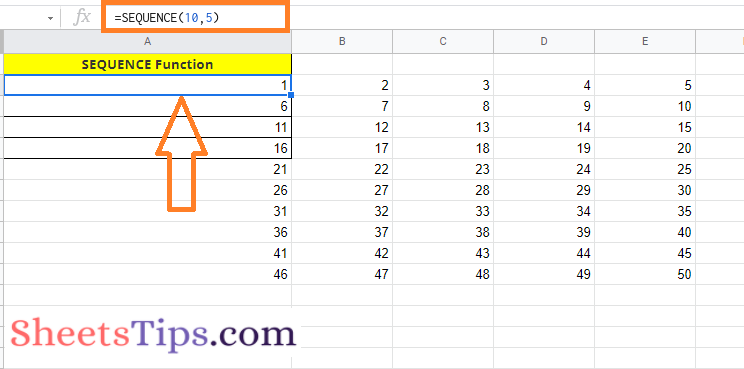### Generating Specific Numbered List Using Sequence Function in Google Sheets

Using the sequence functions in Google Sheets, we can also generate the numbers as per our specification. For example, if we want numbers to be generated in sequential order after the term 100 such as 100, 101, 102, etc., then we can get this done in Google Sheets using the steps given below:

• 1st Step: Open the Google Sheets on your device where you want to generate the numbered list as per your specifications.
• 2nd Step: Now move to the cell where you want to generate the specific sequential number.
• 3rd Step: Enter the formula as =Sequence(5,1,100).
• 4th Step: Press the “Enter” button and you will see the results as shown below.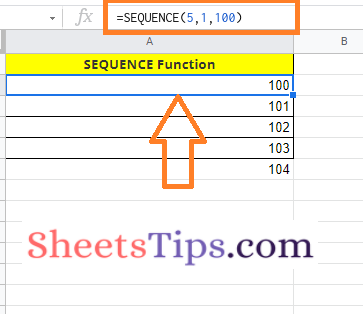### Generating Custom Numbered List Using Sequence Function in Google Sheets

Let us say that I want to generate a numbered list in such an order as 1, 11, 21 which is a kind of customization. Now the steps to generate this in Google Sheets are as follows:

• 1st Step: Open the Google Sheets to generate the custom numbered list.
• 2nd Step: Now on the homepage, move to the cell where you want to generate the customized numbered list.
• 3rd Step: Enter the formula =Sequence(5,1,1,10).
• 4th Step: Press the Enter button and you will see the results as shown in the image below.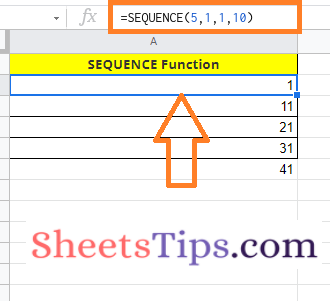### Generating Descending Numbered List Using Sequence Function in Google Sheets

Sequence function in Google Sheets allows us to generate the descending numbered list. The steps to generate the numbered list in reverse order are as follows:

• 1st Step: Open the Google Sheets to generate the descending numbers.
• 2nd Step: Now move to the cell where you want to generate the descending numbers in sequential order.
• 3rd Step: Enter the formula “=sequence(5,1,5,-1)“.
• 4th Step: Press the “Enter” button and you will see the results as shown below.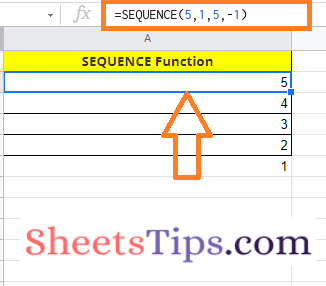### Generating Negative Numbers List Using Sequence Function in Google Sheets

We can also generate the negative numbers list in Google Sheets using the Sequence function. The steps to get this done in Google Sheets are as follows:

• 2nd Step: Now on the homepage, enter the formula “=sequence(5,1,-1,-1)” in which you want to generate the negative numbers.
• 3rd Step: Press the “Enter” button and you will see the results as shown below.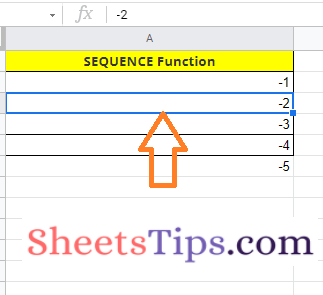### Generating Decimal Numbers List Using Sequence Function in Google Sheets

The steps to generate decimal numbers in sequential order in Google Sheets are as follows: